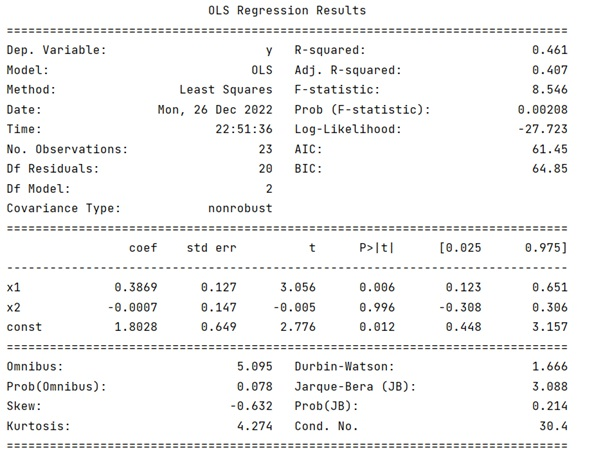# Multiple Linear Regression

Learn about the multiple linear regression in Python?
Submitted by Pranit Sharma, on December 27, 2022

NumPy is an abbreviated form of Numerical Python. It is used for different types of scientific operations in python. Numpy is a vast library in python which is used for almost every kind of scientific or mathematical operation. It is itself an array which is a collection of various methods and functions for processing the arrays.

Linear regression is a statistical method that is used for predictive analysis. Linear regression is used for making predictions for continuous/real values such as sales, salary, age, product price, etc.

Here, we need to find a way to do multiple regression. For this purpose, we will use the statsmodel library which has api module which will be used access, LinearRegression.

Let us understand with the help of an example,

## Python code to demonstrate the example of multiple linear regression

```# Import numpy
import numpy as np

# Import statsmodel
import statsmodels.api as ap

# Create two arrays
a1 = [1,2,3,4,3,4,5,4,5,5,4,5,4,5,4,5,6,5,4,5,4,3,4]
a2 = [[4,2,3,4,5,4,5,6,7,4,8,9,8,8,6,6,5,5,5,5,5,5,5],
[4,1,2,3,4,5,6,7,5,8,7,8,7,8,7,8,7,7,7,7,7,6,5],]

# Defining a function for regression
def fun(x,y):
first = np.ones(len(a1))
for ele in x[1:]:
res = ap.OLS(y, X).fit()
return res

# Calling the function
print(fun(a1,a2).summary())
```

Output: